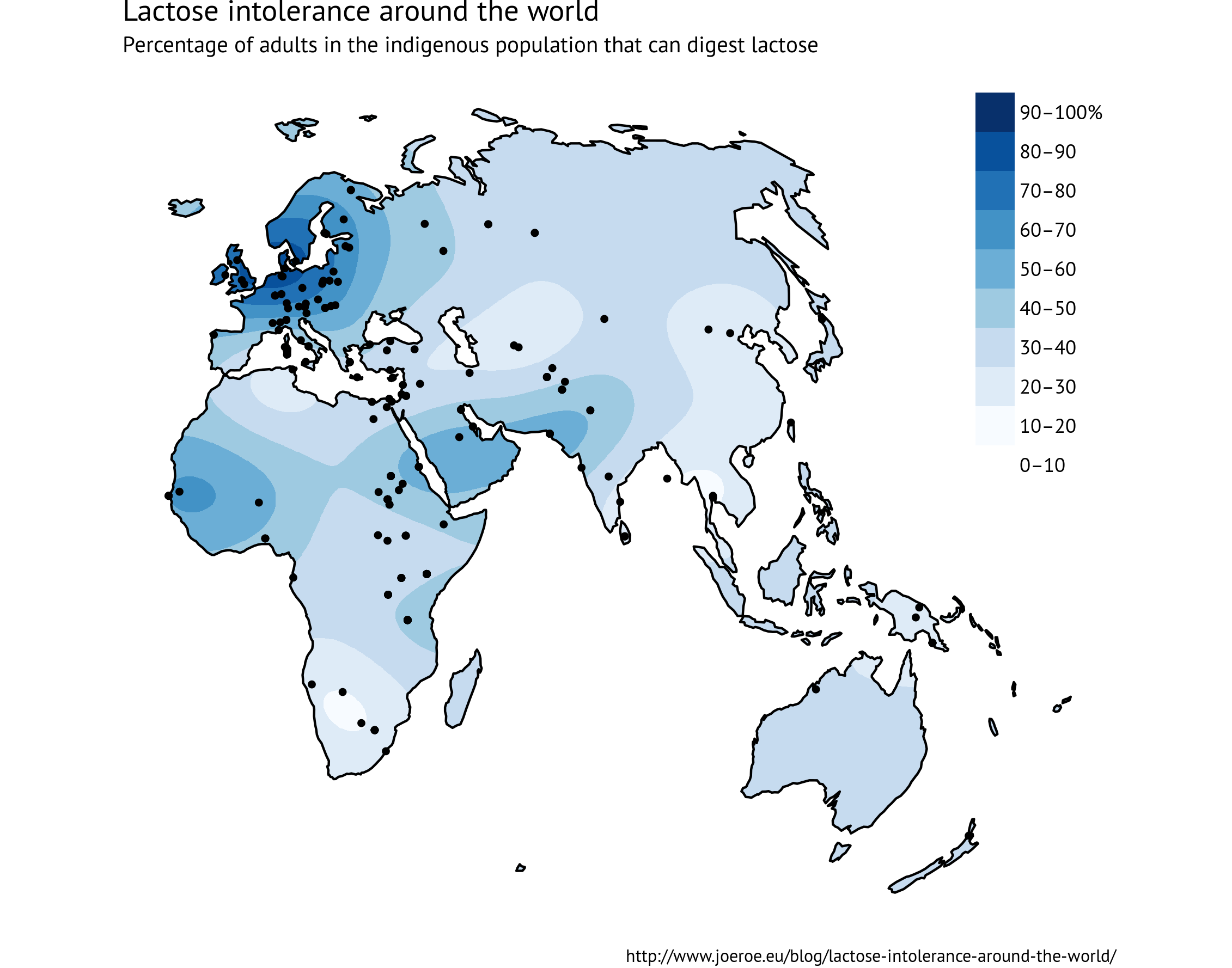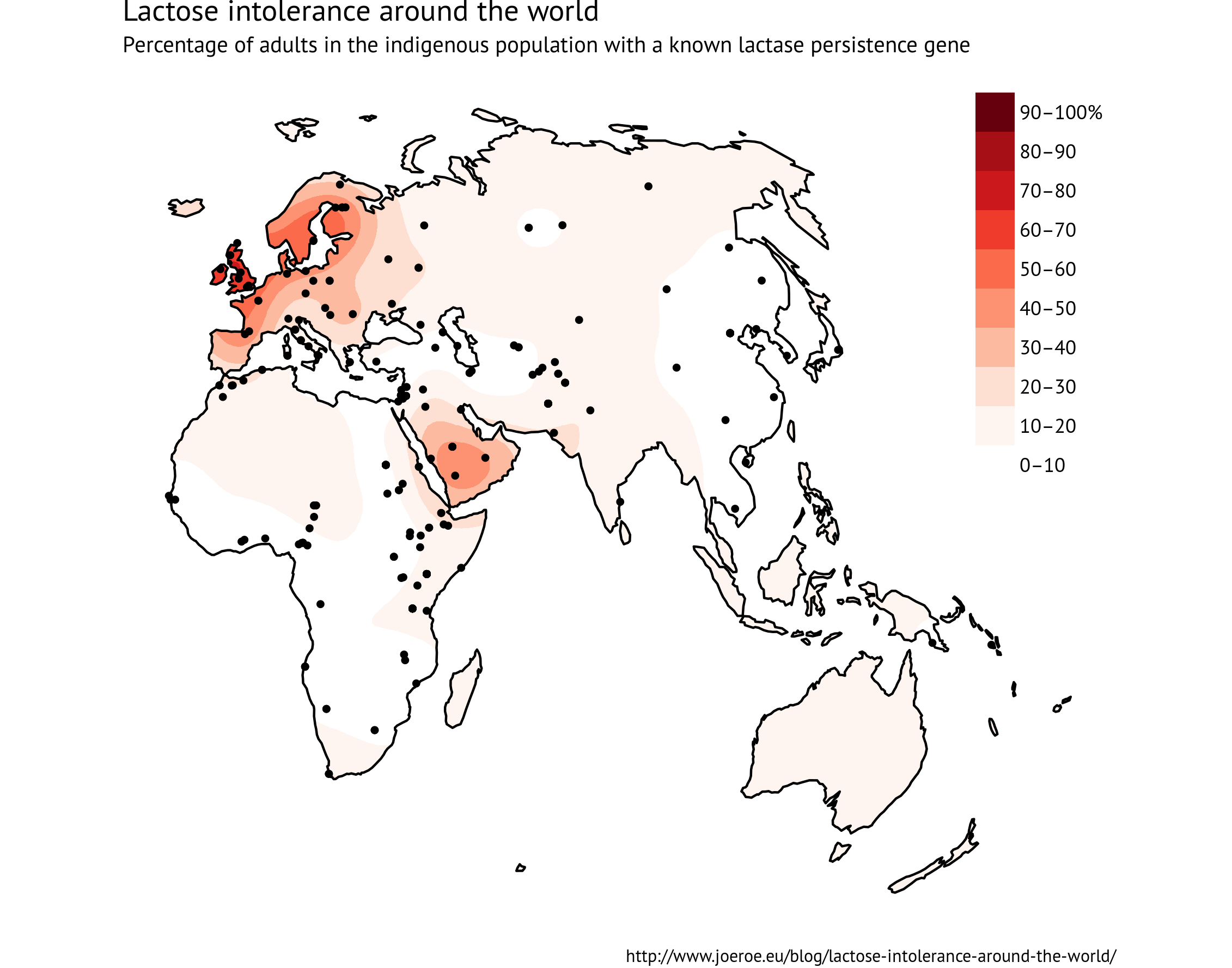# Lactose intolerance around the world

Most of us in the western world take drinking milk for granted, but the ability to digest lactose into adulthood is actually relatively uncommon from a global perspective. It comes from a genetic mutation called lactase persistence, which evolved independently in a few parts of the world in the last 5,000 years, probably as an adaptation to widespread dairying following the Neolithic (or Secondary Products?) Revolution. This makes it one of the clearest examples of gene–culture coevolution we know of, and therefore of great interest to evolutionary anthropologists (Ségurel and Bon 2017).Itan et al. (2010) have done the most comprehensive study of the geographic lactase persistence around the world. Their Global Lactase persistence Association Database (GLAD) includes statistics on the frequency of both the lactose-digesting phenotype and known lactase persistence genotypes in indigenous populations across the Old World. Unfortunately the Americas are not yet represented in the database.

To visualise the distribution of lactase persistence I grabbed the latest data from GLAD and used R to interpolate it onto a map using automated ordinary kriging. This is no different to what Itan et al. did in their 2010 paper, although they used a different method of interpolation. I generated maps for both the phenotype and the genotype: as Itan et al. note, there are significant discrepancies between the two, presumably indicating that we don’t yet know of all the alleles that are associated with lactase persistence.## Source code

The major dependencies are automap and ggplot2. It uses two shapefiles (the Afro-Eurasia-Australian landmass and the Caspian sea), both derived from Natural Earth’s 1:10 physical dataset, that should be simple enough to generate but are included here for convenience:

``````# lp_distribution.R
# Distribution of lactase persistence in the Old World
# Joe Roe <http://www.joeroe.eu/blog/lactose-intolerance-around-the-world>

library("magrittr")
library("rgdal")
library("automap")
library("raster")
library("ggplot2")
library("RColorBrewer")

.[,!sapply(., function(x) all(is.na(x)))] %>%         # Remove empty columns
.[complete.cases(.),] ->                              # and rows
phenotype
coordinates(phenotype) <- c("LONGITUDE", "LATITUDE")
proj4string(phenotype) <- "+proj=longlat"
.[,!sapply(., function(x) all(is.na(x)))] %>%
.[complete.cases(.),] ->
genotype
coordinates(genotype) <- c("LONGITUDE", "LATITUDE")
proj4string(genotype) <- "+proj=longlat"

# Geographic data <http://www.naturalearthdata.com/>

# Reproject lat/long data to a suitable projection (Winkel Tripel)
projection <- CRS("+proj=wintri")
old_world <- spTransform(old_world, projection)
caspian <- spTransform(caspian, projection)
phenotype <- spTransform(phenotype, projection)
genotype <- spTransform(genotype, projection)

# Interpolatation by ordinary kriging (takes a while)
isurface <- expand.grid(x=seq(from=bbox(old_world)["x","min"],
to=bbox(old_world)["x","max"],
by=10000),
y=seq(from=bbox(old_world)["y","min"],
to=bbox(old_world)["y","max"],
by=10000))
coordinates(isurface) <- c("x", "y")
gridded(isurface) <- TRUE
proj4string(isurface) <- proj4string(old_world)

iphenotype <- autoKrige(FREQUENCY.OF.DIGESTORS~1, phenotype, isurface)
igenotype <- autoKrige(SUM.OF.ALL.LP.ASSOCIATED.ALLELES~1, genotype, isurface)

# Clip to land only
iphenotype <- raster(iphenotype\$krige_output)
iphenotype <- mask(iphenotype, old_world)               # Doesn't handle holes in the mask polygon
iphenotype <- mask(iphenotype, caspian, inverse=TRUE)   # So clip the Caspian Sea seperately

igenotype <- raster(igenotype\$krige_output)

# Map of lactose digestors
c_iphenotype <- as.data.frame(cut(iphenotype, seq(0, 1, by=0.1)), xy=TRUE)
c_iphenotype\$layer <- factor(c_iphenotype\$layer, levels=1:10,
labels=c("0–10", "10–20", "20–30", "30–40", "40–50",
"50–60", "60–70", "70–80", "80–90", "90–100%"))

ggplot() +
coord_fixed() +
geom_raster(data=c_iphenotype, aes(x=x, y=y, fill=layer)) +
geom_polygon(data=fortify(old_world), aes(x=long, y=lat, group=group), colour="black", fill=NA) +
geom_point(data=data.frame(phenotype), aes(x=LONGITUDE, y=LATITUDE), size=1) +
scale_fill_manual(values=c("#FFFFFF", brewer.pal(9, "Blues")), drop=FALSE,
na.translate=FALSE, name=NULL, guide=guide_legend(reverse=TRUE)) +
labs(title="Lactose intolerance around the world",
subtitle="Percentage of adults in the indigenous population that can digest lactose",
caption="http://www.joeroe.eu/blog/lactose-intolerance-around-the-world/") +
theme_void(base_size=11, base_family="PT Sans") +
theme(legend.justification=c(1,1), legend.position=c(1,1),
plot.caption=element_text(size=rel(0.7))) ->
p_iphenotype

# Map of LP genes
c_igenotype <- as.data.frame(cut(igenotype, seq(0, 1, by=0.1)), xy=TRUE)
c_igenotype\$layer <- factor(c_igenotype\$layer, levels=1:10,
labels=c("0–10", "10–20", "20–30", "30–40", "40–50",
"50–60", "60–70", "70–80", "80–90", "90–100%"))

ggplot() +
coord_fixed() +
geom_raster(data=c_igenotype, aes(x=x, y=y, fill=layer)) +
geom_polygon(data=fortify(old_world), aes(x=long, y=lat, group=group), colour="black", fill=NA) +
geom_point(data=data.frame(genotype), aes(x=LONGITUDE, y=LATITUDE), size=1) +
scale_fill_manual(values=c("#FFFFFF", brewer.pal(9, "Reds")), drop=FALSE,
na.translate=FALSE, name=NULL, guide=guide_legend(reverse=TRUE)) +
labs(title="Lactose intolerance around the world",
subtitle="Percentage of adults in the indigenous population with a known lactase persistence gene",
caption="http://www.joeroe.eu/blog/lactose-intolerance-around-the-world/") +
theme_void(base_size=11, base_family="PT Sans") +
theme(legend.justification=c(1,1), legend.position=c(1,1),
plot.caption=element_text(size=rel(0.7))) ->
p_igenotype

# Export plots
ggsave("./output/lp_phenotype.png", plot=p_iphenotype)
ggsave("./output/lp_genotype.png", plot=p_genotype)
``````

Tags:

Updated: